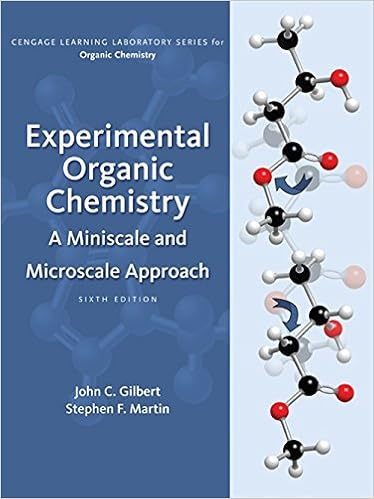Sample Lab - Short Answer Sample Lab Experiment 1 Lab...

• Lab Report
• 3

This preview shows page 1 - 3 out of 3 pages.

We have textbook solutions for you!
The document you are viewing contains questions related to this textbook.The document you are viewing contains questions related to this textbook.
Chapter 14 / Exercise 1
Experimental Organic Chemistry: A Miniscale & Microscale Approach
Gilbert/MartinExpert Verified
Short Answer Sample Lab Experiment 1 Lab Results 1. Record the maximum temperature reached by the contents of the Erlenmeyer flask. 2. 25.5 Data Analysis 1. Did a reaction happen in the Erlenmeyer flask? How can you tell?
Experiment 2 Lab Results 1. Fill in the table below with the data you collected during the experiment. The first line is filled out for you as an example. 2. Volume of Water Added (mL) 3. Pressure Inside the Flask (atm) 4. 0 5. 1.00 6. 20 7. 1.15 8. 40 9. 1.36 10. 60 11. 1.67 12. 80 13. 2.14 14. 100 15. 3.0
We have textbook solutions for you!
The document you are viewing contains questions related to this textbook.The document you are viewing contains questions related to this textbook.
Chapter 14 / Exercise 1
Experimental Organic Chemistry: A Miniscale & Microscale Approach
Gilbert/MartinExpert Verified
Data Analysis 1. Calculate the values in the table below using the data you recorded during the experiment. Subtract the volume of water added from the total volume of the flask to determine the volume of air. The first line is filled out for you as an example. 2. Volume of Air in the Flask (mL) 3. 1 / Pressure (atm –1 ) 4. 150 mL 5. 1 6. 130mL 7. 2 8. 110mL 9. 3 10. 90mL 11. 4 12. 70mL 13. 5 14. 50mL 15. 6 16. 17. Draw a graph of 1 / pressure ( y -axis) versus volume of air ( x -axis). Click on the
•••